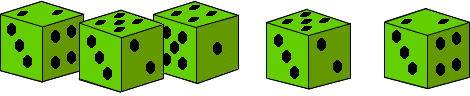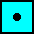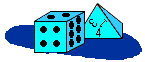Objective 14 Make the connection between the number of faces for various dice, and the probability of a single event.
Problem
 Stacey noticed that the theoretical and experimental probability for rolling a 2 on her 6-sided die were the same after rolling 60 times. If the experimental probability of rolling a 2 is one-third, how many times did she roll a 2? How many twos are on her die?Manipulative
Materials: one regular six-sided die, one regular four-sided die, one regular eight-sided die.
 Roll a 6-sided die 60 times. How manys did you roll? Repeat with an 8-sided die. Repeat with a 4-sided die. With which die did you roll the most ones? the least?• Calculate the theoretical probability of rolling a one for each die. Which die has the greatest, and which has the least theoretical probability of rolling a '1'?
Game
 Materials: one regular 4-sided die, one regular 6-sided die, one regular 8-sided die, base ten blocks. On a turn select a die (4, 6 or 8 sides) and and roll it. Take the same number of single base ten blocks as the number rolled (regroup if possible). If your total number of blocks is a number divisible evenly by 5, score one point. If your total number also divides by 10 evenly, score a second point. Players keep rolling and taking blocks until one player has 50 blocks exactly. Whoever has the greatest point score wins. Adaptations: Make your own 4 and 6-sided dice such that you can choose dice which increase your odds of rolling small numbers. This should make it easier to accumulate multiples of 5 and 10.